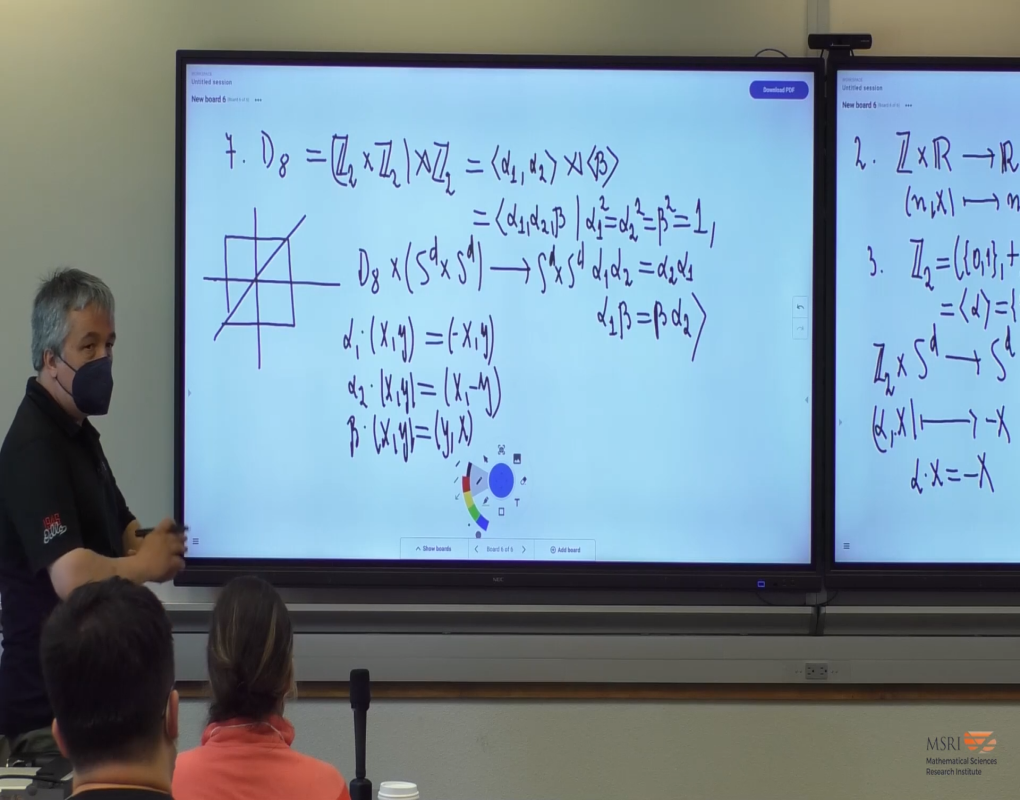### Videos

#### Topological Methods For The Discrete Mathematician: Using Equivariant Topology Pt I

##### Presenter
July 25, 2022##### Abstract
Various classes of problems from discrete and convex geometry, mathematical mechanics, algorithm complexity theory and approximation theory can be transformed into challenging, and often unsolved problems in equivariant topology. The focus of our lecture series is understanding where these topological problems come from and then demonstrating some of the diverse methods of algebraic topology which are critical to study and subsequently solve them.